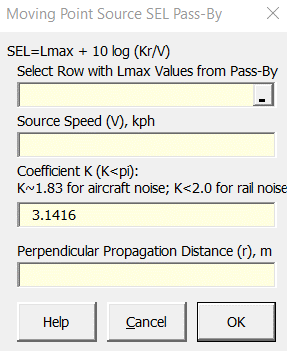### Strutt Help

Moving Point Source SEL Pass-By    1/1

Strutt|Propagation|SEL from Moving Point Source inserts the Sound Exposure Level (SEL) from a moving point source into the active row of the worksheet.The SEL from a moving point source is calculated by:

SEL = L_max+10log_10((Kr)/V), where K <= pi

For an omnidirectional source, K=pi
A value of K = 1.83 is suggested for aircraft noise. For diesel locomotives, the directivity characteristics of the source lead to a maximum value of 2.0 for K.
V is the velocity of moving point source.
r is the distance to the receiver.

References:

• Transportation Noise Reference Book p2/8